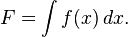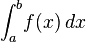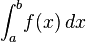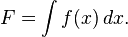# How do I write an integral from a to b in latex?

• LaTeX
phosgene
As the title suggests, I can only see how to write an integral like:But how would I write an integral like the following?## Answers and Replies

Homework Helper
As the title suggests, I can only see how to write an integral like:But how would I write an integral like the following?Put a subscript and a superscript on the \int, e.g. \int_{a}^{b}

phosgene
Oh, argh! Why didn't I think of that? Anyway, thanks :)

Staff Emeritus
Homework Helper
Homework Helper
Gold Member
Also, if you see an example of what you want to do, just right click on it and select to show math as Tex commands to see the code. You can copy/paste from that.

whatlifeforme
As the title suggests, I can only see how to write an integral like:But how would I write an integral like the following?how do i make the size and font look like that iwth latex? is that latex or a link to an image?

how do i make the size and font look like that iwth latex? is that latex or a link to an image?

It's just an image.

Mentor
Here's the actual LaTeX for phosgene's two integrals:

$$F = \int {f(x) dx}$$

$$\int_a^b {f(x) dx}$$

The right-click trick should work on those. Or control-click if you're using a Mac, like I am.

Mentor
As the title suggests, I can only see how to write an integral like:But how would I write an integral like the following?Put a subscript and a superscript on the \int, e.g. \int_{a}^{b}

As long as the subscripts/superscripts are single characters, as in the above, you can omit the braces around the sub-/superscript. The following will render exactly the same:
\int_a^b

When there are two or more characters (e.g. 2x, -3, etc.) you need the braces around the entire expression, as in this example:
\int_{-2}^{3x}

whatlifeforme
Here's the actual LaTeX for phosgene's two integrals:

$$F = \int {f(x) dx}$$

$$\int_a^b {f(x) dx}$$

The right-click trick should work on those. Or control-click if you're using a Mac, like I am.

mine looks smaller for some reason.

$\int_a^b {f(x) dx}$

tex $$\int_a^b {f(x) dx}$$
itex $\int_a^b {f(x) dx}$
itex with \displaystyle $\displaystyle\int_a^b {f(x) dx}$